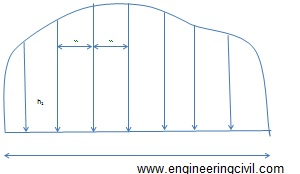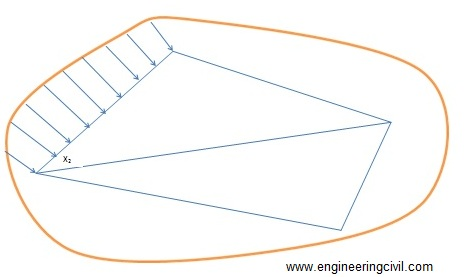Awarded as the best online publication by CIDC

# Student Corner

Instrumental methods-use of planimeter

Keywords: planimeter, Computation of area, zero circle
Instrumental methods are one of the important technique uses to determine area of a plot. The other methods for area computation are using field notes and from previously plotted plan. We have already discussed them here.
Computation of areas and volumes | Computation of Area from Plan

Significance of Planimeter in Area computation
The planimeter gives more accurate (within one percent) results compared to the other methods except by direct calculation from the field notes. It is used for determining the areas of figures plotted to scale, especially when the boundaries are irregular. Example for such plots are, plot with irregular boundary and plot having a river as boundary on one side.

Computation of Area from Plan

Keywords: graphical methods, area computation, mid ordinate rule, trapezoid rule, Simpson’s rule, Parabolic rule

This article discusses the second method for computation of area (i.e., from previously plotted plan). You can read the first method ( computation of area using field notes) here.

Several methods can be used to determine the areas from plans. These methods are generally classified as graphical methods and instrumental methods

1) Graphical methods
These methods use the measured data from the existing plan. The areas may be calculated for the area as a whole or the areas is found by adding the areas of the skeleton and the area of the irregular strip between the skeleton and the boundary.

a) Entire areas
Generally used methods to calculate the area as whole is enumerated as follows:

i) By division of the area into triangles
In this method the total area is divided into a number of triangles. The base and altitude of each triangle are, measured and its area is found. When the boundaries are irregular, straight lines termed as ‘give and take’ or ‘equalizing lines’ replace them. These lines are so drawn that they exclude as much as they include as shown in figure.

ii) By division of the area into squares
This method is explains by figure. A piece of tracing paper is divided into squares of equal areas. This is place over the drawing. The total number of complete squares and fractions of squares formed along the boundaries are estimated in terms of full squares. The area of the plan is equal to the number of square’s multiplies by the area of one square.

iii) By division of the area into trapezoids
A number of equidistant parallel lines are drawn on a tracing paper and the tracing paper is placed over the plan so that the area is exactly enclosed between two parallel lines. Then the plotted area is divided into a number of strips. The curved ends of which are replaced by perpendicular ‘give and take’ lines. The sum of the base length of the rectangles thus obtains, multiplied by the common distance between the parallel lines gives the area of the plan.

b) Areas along boundaries
The areas enclosed between the adjacent survey lines and the curved boundaries are determined by using ordinated. The survey line is divided into a number of equal parts and the ordinate at each point is drawn and scaled. The area can be calculated by several methods as described below:

i) Mid ordinate rule
Ordinates are measured at the midpoint of each division.

Area= (h1+h2+h3+……+hn) L/n

Area= (h1+h2+h3+…..+hn) d

Where
h1,h2,h3,h4…..,hn= ordinates at the midpoint of each division
L= length of the base line
n= the number of equal parts into which the baselines is divided
d= the common distance between the ordinates=L/nFigure 1: Illustration of mid ordinate rule

ii) Trapezoid rule
This rule is more accurate than the mid-ordinate and the average ordinate rules. To apply this method, the whole area has to be divided into a number of trapezoids. The rule can be stated as ‘to the sum of the first and last ordinates, ass twice the sum of the intermediate ordinates. Then multiply the total sum with the common distance between the ordinates. The one half of this product gives the required area’

Area=d/2 {O0+On+2(O1+O2+….+On)}

When the baseline cuts the boundary at one or both ends, then O0 or On are zero. In that case O0 and On becomes zero in the equation above.

The trapezoid rule assumes that the boundaries between the ends of the ordinates are straight lines. The derivation of the formula is simple and can be tried out.

iii) Simpson’s rule or Parabolic rule
The rule may be stated as ‘to the sum of the first and last ordinates, add twice the sum of the remaining odd ordinates and four times the sum of all the even ordinates. Multiply the total sum thus obtained by one-third f the common distance between the ordinates. This gives the area requires’

Area-d/3 {O0+On+2 (O2+O4+O6+….) 4 (O1+O3+O5…)}

This method requires that there be an even number of divisions of the area. If there is odd number of divisions, the area of the last division must be calculated separately and added to the result obtained by applying the Simpson’s rule to the remaining even divisions.It is to be noted that an odd number of ordinates corresponds to an even number of divisions.

The second method to calculate area from a previously plotted plan (i.e. instrumental methods) is discussed here.

Computation of areas and volumes

Keywords: computation of area, computation volume, area calculation of irregular boundaries

This article discusses the methods which are used to determine the areas of lands and the volumes of earthwork (either cutting or filling). These methods find application in determining the areas of existing plots. Volume determination is useful to determine the volumes of cutting through elevated areas and volume of embankment for the purpose of laying routes through low lying areas.

Computation of areas
Area of land means the area as projected on a horizontal plane. The important point is,it is not the actual area of surface.
1) To determine the area of a plot with straight boundaries, the plot is then subdivided into areas having straight boundaries (i.e. triangles, rectangles or trapezium) and the area calculated using appropriated formulas for this subdivided plots.

2) To determine areas with irregular boundaries, the plot is then subdivided into areas (of regular polygons) leaving the strip of irregular land along the boundaries whose area is determined separately and added to the calculated of regular polygons.

Area is determined by two methods namely:
1. By direct use of field notes
2. From previously plotted plan

The first method for the computation of areas, i.e., the area determination using field notes is explained as follows:

Area determined by directly using the field notes
To determine the area using this method, it is not necessary to draw the whole plan to scale. Because the accuracy of the work is solely depend on the accuracy of the fieldwork, which is already done. In doing the fieldwork, as much of the whole area as possible is to be included for accuracy. The survey lines shouldlie down carefully for this.This is shown in figure 1 as follows:Figure 1:  Planning of the survey lines for the determination of area

The calculation of the plot area consists of two parts namely:
i. Calculation of the areas of the skeletons
ii. Calculations of the area enclosed between the surveys lines of the skeleton and the irregular boundaries.

Determination of the areas of the skeletons
a. By geometry
The areas of the triangles forming skeletons may be calculated by the following formulae:
1) Area of triangle= ?[s(s-a)(s-b)(s-c)]
Where a, b and c denotes the sides of the triangle
Semi-perimeter, s = (a+b+c)/2

2) Area of triangle=bh/2
Where b is the length of any side and h is the perpendicular distance from this side.

b) Area by co-ordinates
This method is used in the case of traverse survey. In this method of survey, first, a set of points well distributed in the area to be measured is selected. These points are joined by survey lines from which perpendicular offsets may be taken to the boundary. The measurements to be taken in such a traverse survey are essentially the distance of the lines connecting the points and the angle between successive lines or the bearings of the lines (bearing is the angle between the north direction and the line, measures clockwise from north).

The step wise procedures are given below:

• Arrange the co-ordinates in an order.
• Find the sum of the product of the co-ordinates joined by full lines, and the sum of the products of the co-ordinates joined by broken lines.
• Find the difference between the sums
• This difference is equal to twice the area of the traverse.
• Half this difference =area of the traverse

Determination of the area enclosed between the surveys lines of the skeleton and the irregular boundaries
To determine the areas of strips of land along the foundation, the adjoining survey line is decided into intervals and the offset to the boundary is measured at each point. The areas of the irregular strips may now be calculated by multiplying the mean of each successive pair of adjacent offsets by the distance between them as shown in figure 2.

O1= offset at chainage X1

O2= Offset at chainage X2

Mean offset= (O1+O2)/2

Distance between the offsets = X2-X1

Area of the portion between the skeleton X2 and X1 and the boundary = {(O1+O2)/2}(X2-X1)Figure 2: Determination of the area along boundaries

The second method for the computation of area (i.e. computation of area from previously plotted plan) is discussed here.

What are various Types of Cement?

Cement is a construction material which is used as a binder. It binds other construction material aggregate and sand together. Cement exhibits different properties if we change the chemical composition.  Earlier the different types of cement was found by only varying the relative proportion of the oxide composition.As time advances, new materials like additives to the clinker at the time of grinding, use of new raw materials resulted in production of new variety of cement.The various types of cements as per Indian Standards are-

Successful Project Management in the Construction Industry Definition & Characteristic Activities

By
Sreenivasa Hassan Jayaram
CEO & Founder, Venkatarjuna Construction & Consulting, Bangalore, Karnataka, India

Definition: Project Management is the creation and scientific discipline of changing over a thought into outcome effectively and professionally.

Project Management gives the clear forecast of Planning, Execution and Communication.

Characteristic Project Management Activities:

• Approval
• Plan and schedule
• Distribution of responsibility
• Direct to accomplishments
• Know expectations
• Resolve Issues
• Track Project
• Communicate to stakeholders